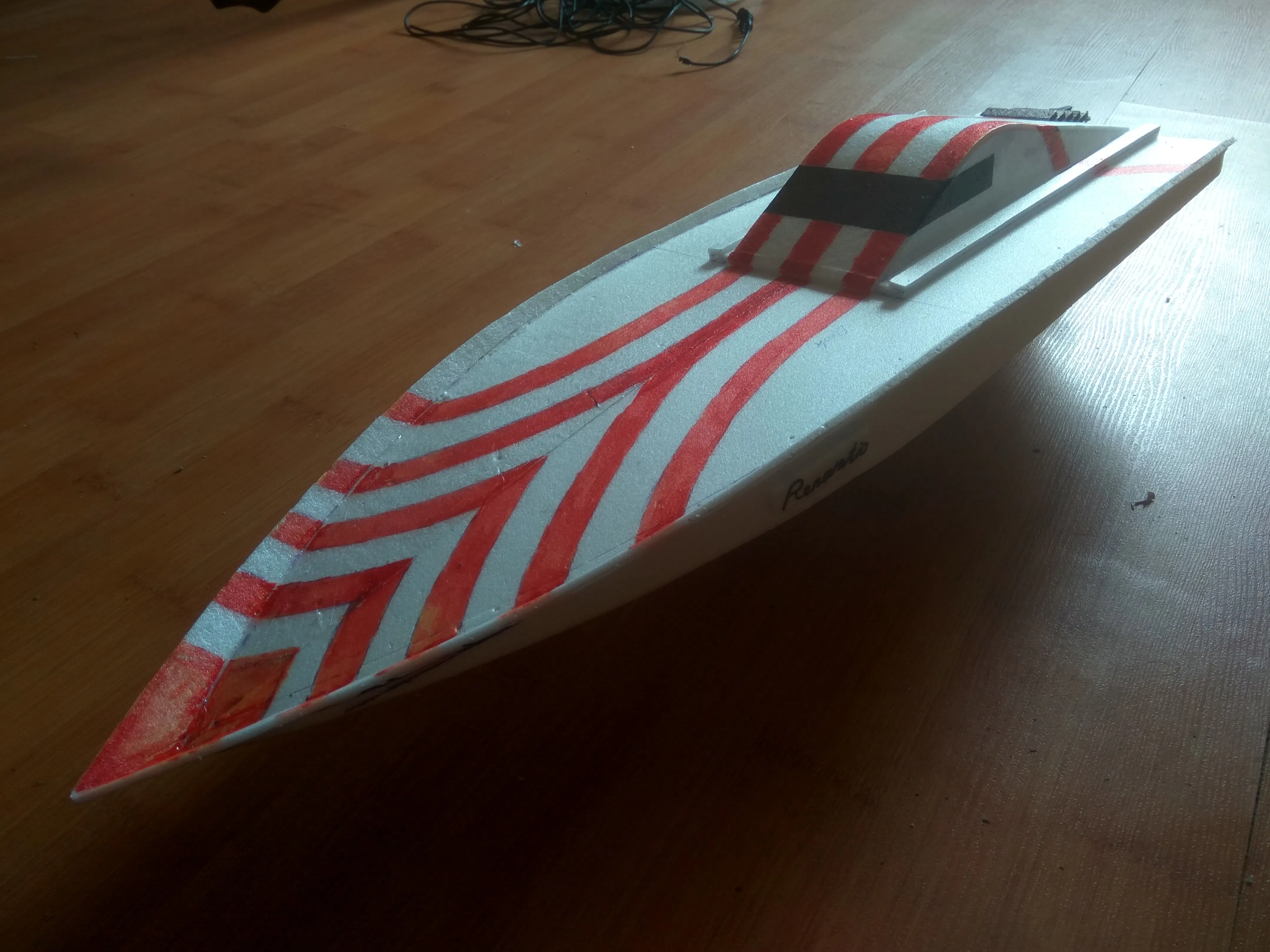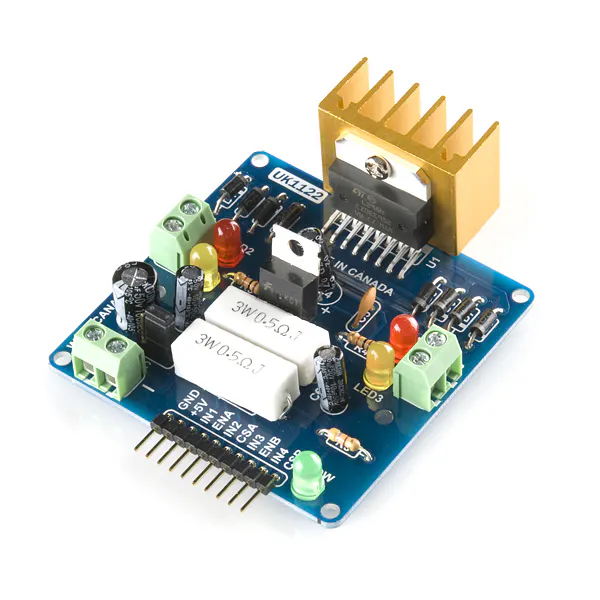Published

# Arduino Bluetooth Boat

A DIY Bluetooth control boat made from Styrofoam and an Arduino Nano.## Things used in this project

### Hardware componentsArduino Nano R3
×1SparkFun Dual H-Bridge motor drivers L298
×1
 Bluetooth Low Energy (BLE) Module (Generic) I use HC-06
×1
 Servos (Tower Pro MG996R)
×1

### Software apps and online servicesArduino IDE for coding
 Android Arduino Bluetooth RC Car

## Schematics

### Roat

two channels on motor driver connected in parallel because i use motor 370 class and in parallel I increased the maximum current to 4A

## Code

### bluetooth_test.ino

C/C++
```#include <Servo.h>
Servo myservo;

int val;
int LED = 13;
int IN4 = 4; // Input3    5
int IN3 = 5;
int IN2 = 7;
int IN1 = 6;
int k = 0;
void setup()
{
Serial.begin(9600);
pinMode(LED, OUTPUT);
pinMode (IN4, OUTPUT);
pinMode (IN3, OUTPUT);
pinMode (IN2, OUTPUT);
pinMode (IN1, OUTPUT);
myservo.attach(10);
}

void loop()
{
if (Serial.available())
{

if (k == 0)
{
myservo.write(90);  // (1    )
k++;
}
//   "F"   ( w)
if (val == 'X')
{
myservo.write(90);
}
if (val == 'x')
{
myservo.write(90);
}
if (val == 'F')
{
digitalWrite (IN4, HIGH);
digitalWrite (IN3, LOW);
digitalWrite (IN2, HIGH);
digitalWrite (IN1, LOW);
}
//   "S"
if ( val == 'S')
{
digitalWrite (IN3, LOW);
digitalWrite (IN4, LOW);
digitalWrite (IN2, LOW);
digitalWrite (IN1, LOW);
}
//   "B"
if ( val == 'B')
{
digitalWrite (IN4, LOW);
digitalWrite (IN3, HIGH);
digitalWrite (IN2, LOW);
digitalWrite (IN1, HIGH);
}
//
if ( val == 'L')
{
myservo.write(50);
//  myservo.write(90);
}
//
if ( val == 'R')
{
myservo.write(130);
//  myservo.write(90);
}

//  45 +

//   "G"
if (val == 'G')
{
digitalWrite (IN4, HIGH);
digitalWrite (IN3, LOW);
digitalWrite (IN2, HIGH);
digitalWrite (IN1, LOW);
myservo.write(50);
delay(1000);
myservo.write(90);
}

//   "I"
if ( val == 'I')
{
digitalWrite (IN4, HIGH);
digitalWrite (IN3,LOW);
digitalWrite (IN2, HIGH);
digitalWrite (IN1, LOW);
myservo.write(130);
delay(1000);
myservo.write(90);
}
//   ""
if ( val == 'H')
{
digitalWrite (IN4, LOW);
digitalWrite (IN3, HIGH);
digitalWrite (IN1, HIGH);
digitalWrite (IN2, LOW);
myservo.write(130);
delay(1000);
myservo.write(90);
}
//   "J"
if ( val == 'J')
{
digitalWrite (IN4, LOW);
digitalWrite (IN3, HIGH);
digitalWrite (IN1, HIGH);
digitalWrite (IN2, LOW);
myservo.write(50);
delay(1000);
myservo.write(90);
}
}
}
```